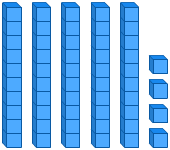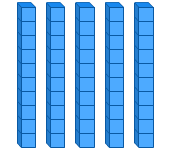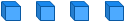# Place Value Models - Tens and Ones

Rate 0 stars Common Core
Quiz size:
Message preview:
Someone you know has shared quiz with you:

To play this quiz, click on the link below:

https://www.turtlediary.com/quiz/place-value-models-tens-and-ones.html

Hope you have a good experience with this site and recommend to your friends too.

Login to rate activities and track progress.
Login to rate activities and track progress.

Consider the model given below.Let's identify the number represented by this model.

First, we count the tens.There are 5 tens.

Now, count the ones.There are 4 ones.

Now, put the values of tens and ones in a place value chart.

 Tens Ones 5 4

So, the number represented by the model is 54.

ds

A B C D E F G H I J K L M N O P Q R S T U V W X Y Z

### Help

##### Remember :

The smallest number is the one that comes first while counting.

##### Solution :

To arrange the given numbers in order from smallest to greatest, find the smallest number among all the given numbers.

21,27,23

21 is the smallest number.